Hits: 5

# Binary Search Tree (BST)

#### In this tutorial, you will learn how Binary Search Tree works. Also, you will find working examples of Binary Search Tree in Python.

Binary search tree is a data structure that quickly allows us to maintain a sorted list of numbers.

• It is called a binary tree because each tree node has a maximum of two children.
• It is called a search tree because it can be used to search for the presence of a number in `O(log(n))` time.

The properties that separate a binary search tree from a regular binary tree is

1. All nodes of left subtree are less than the root node
2. All nodes of right subtree are more than the root node
3. Both subtrees of each node are also BSTs i.e. they have the above two propertiesA tree having a right subtree with one value smaller than the root is shown to demonstrate that it is not a valid binary search tree

The binary tree on the right isn’t a binary search tree because the right subtree of the node “3” contains a value smaller than it.

There are two basic operations that you can perform on a binary search tree:

The algorithm depends on the property of BST that if each left subtree has values below root and each right subtree has values above the root.

If the value is below the root, we can say for sure that the value is not in the right subtree; we need to only search in the left subtree and if the value is above the root, we can say for sure that the value is not in the left subtree; we need to only search in the right subtree.

Algorithm:

``````If root == NULL
return NULL;
If number == root->data
return root->data;
If number < root->data
return search(root->left)
If number > root->data
return search(root->right)``````

Let us try to visualize this with a diagram.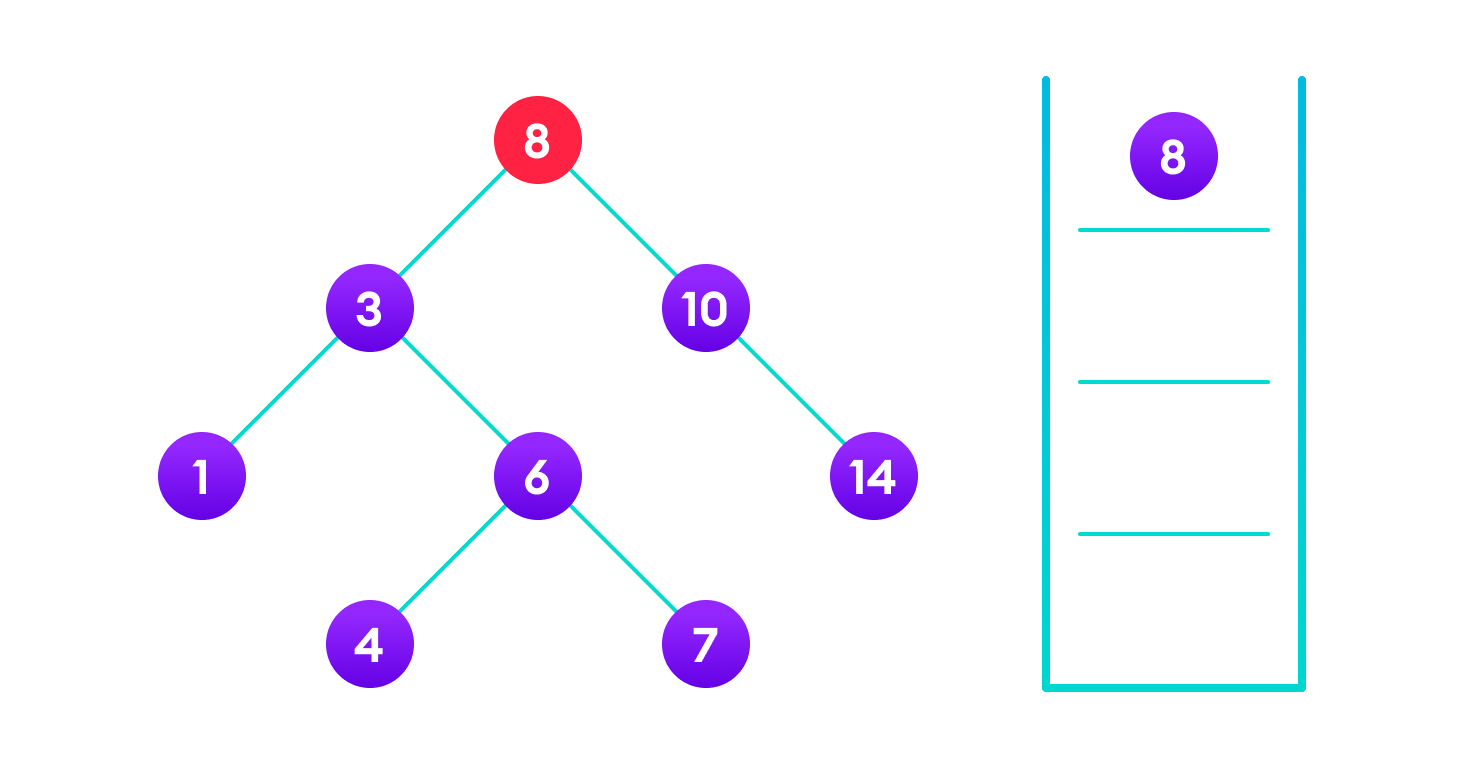4 is not found so, traverse through the left subtree of 8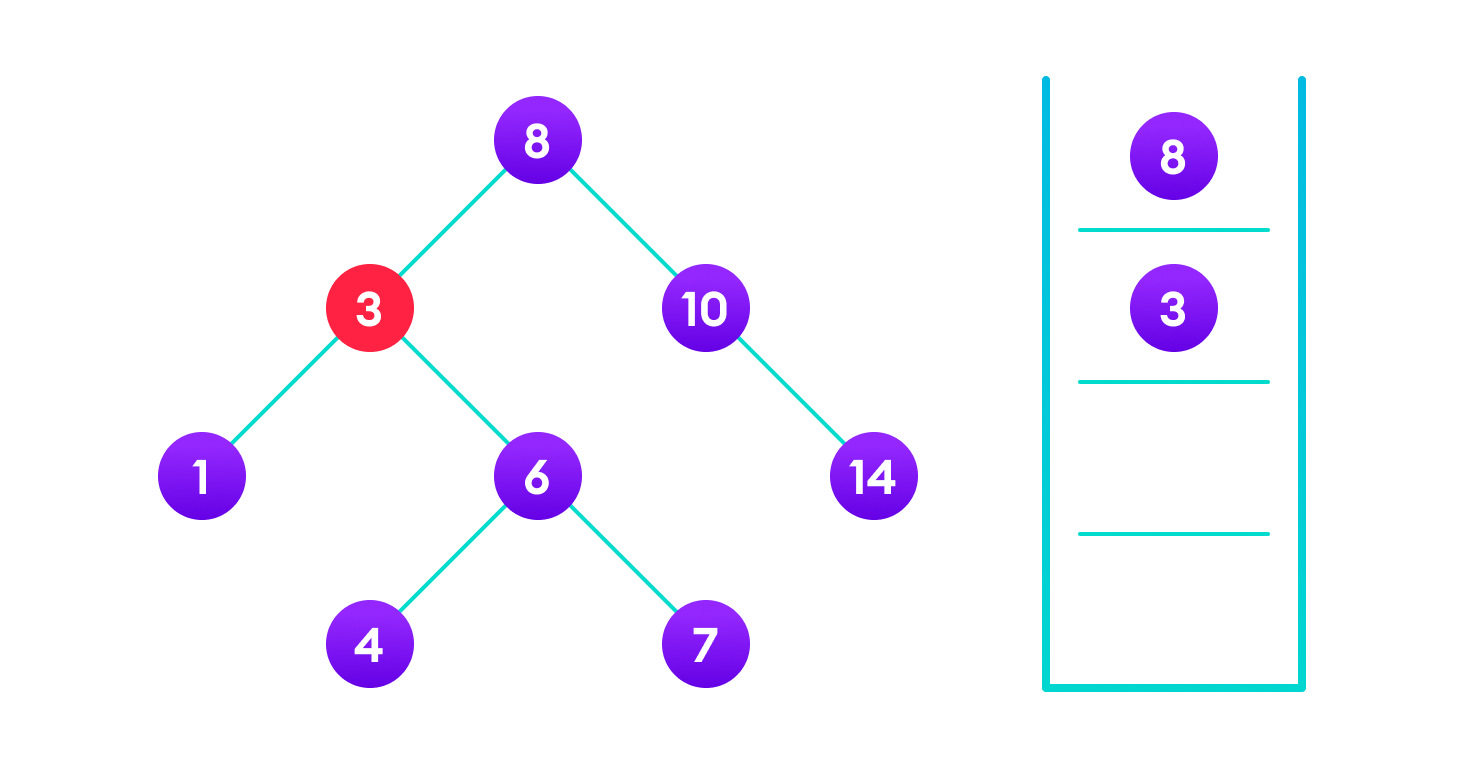4 is not found so, traverse through the right subtree of 3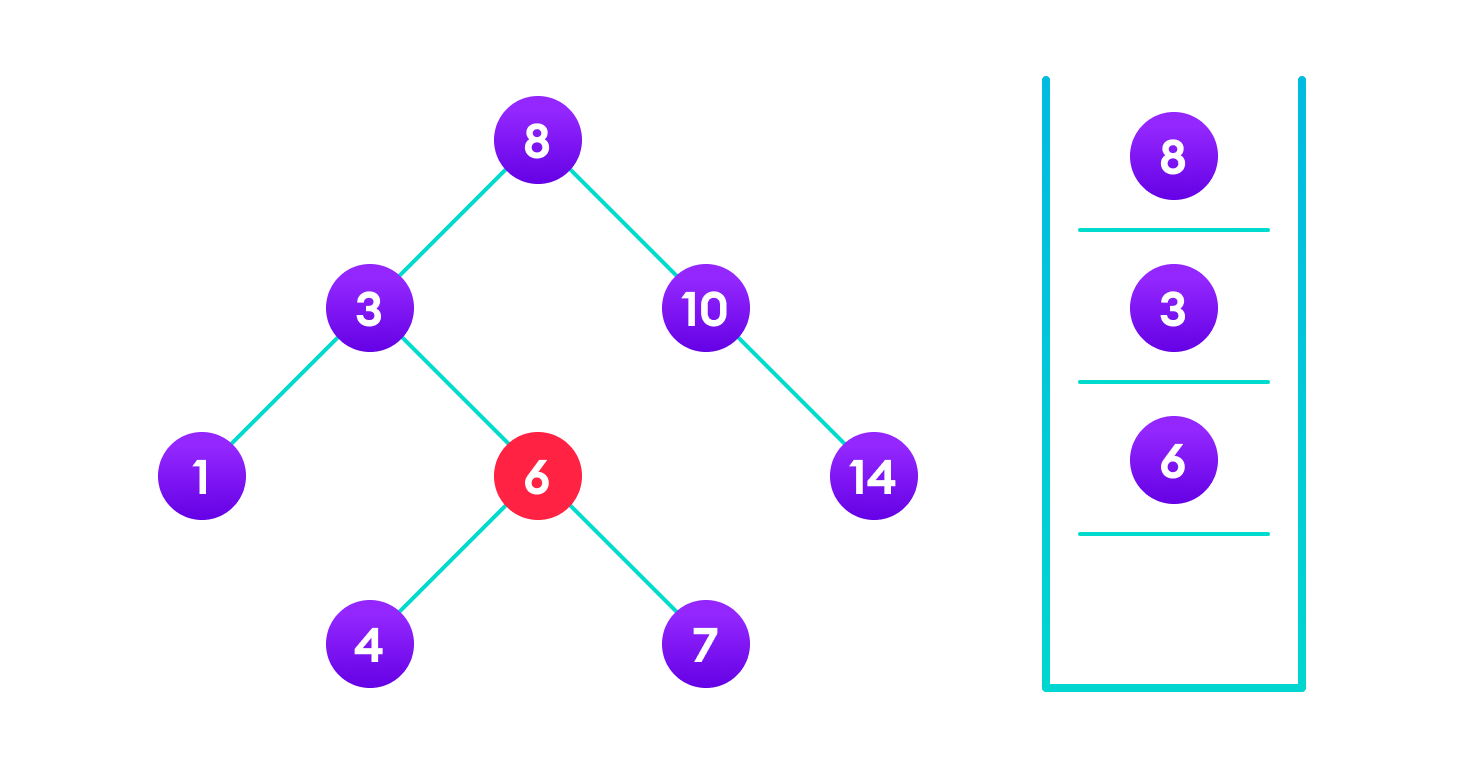4 is not found so, traverse through the left subtree of 64 is found

If the value is found, we return the value so that it gets propagated in each recursion step as shown in the image below.

If you might have noticed, we have called return search(struct node*) four times. When we return either the new node or NULL, the value gets returned again and again until search(root) returns the final result.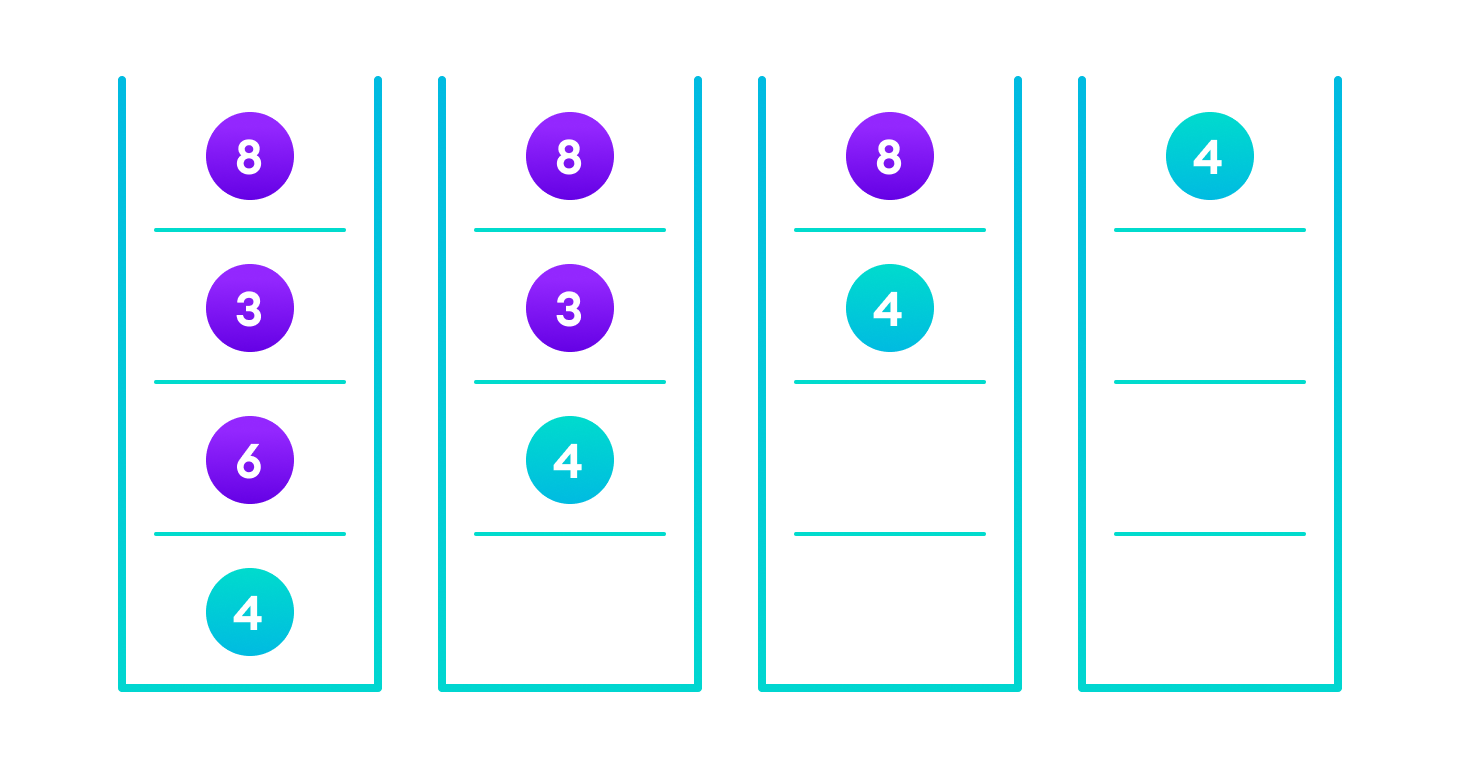If the value is found in any of the subtrees, it is propagated up so that in the end it is returned, otherwise null is returned

If the value is not found, we eventually reach the left or right child of a leaf node which is NULL and it gets propagated and returned.

## Insert Operation

Inserting a value in the correct position is similar to searching because we try to maintain the rule that the left subtree is lesser than root and the right subtree is larger than root.

We keep going to either right subtree or left subtree depending on the value and when we reach a point left or right subtree is null, we put the new node there.

Algorithm:

``````If node == NULL
return createNode(data)
if (data < node->data)
node->left  = insert(node->left, data);
else if (data > node->data)
node->right = insert(node->right, data);
return node;``````

The algorithm isn’t as simple as it looks. Let’s try to visualize how we add a number to an existing BST.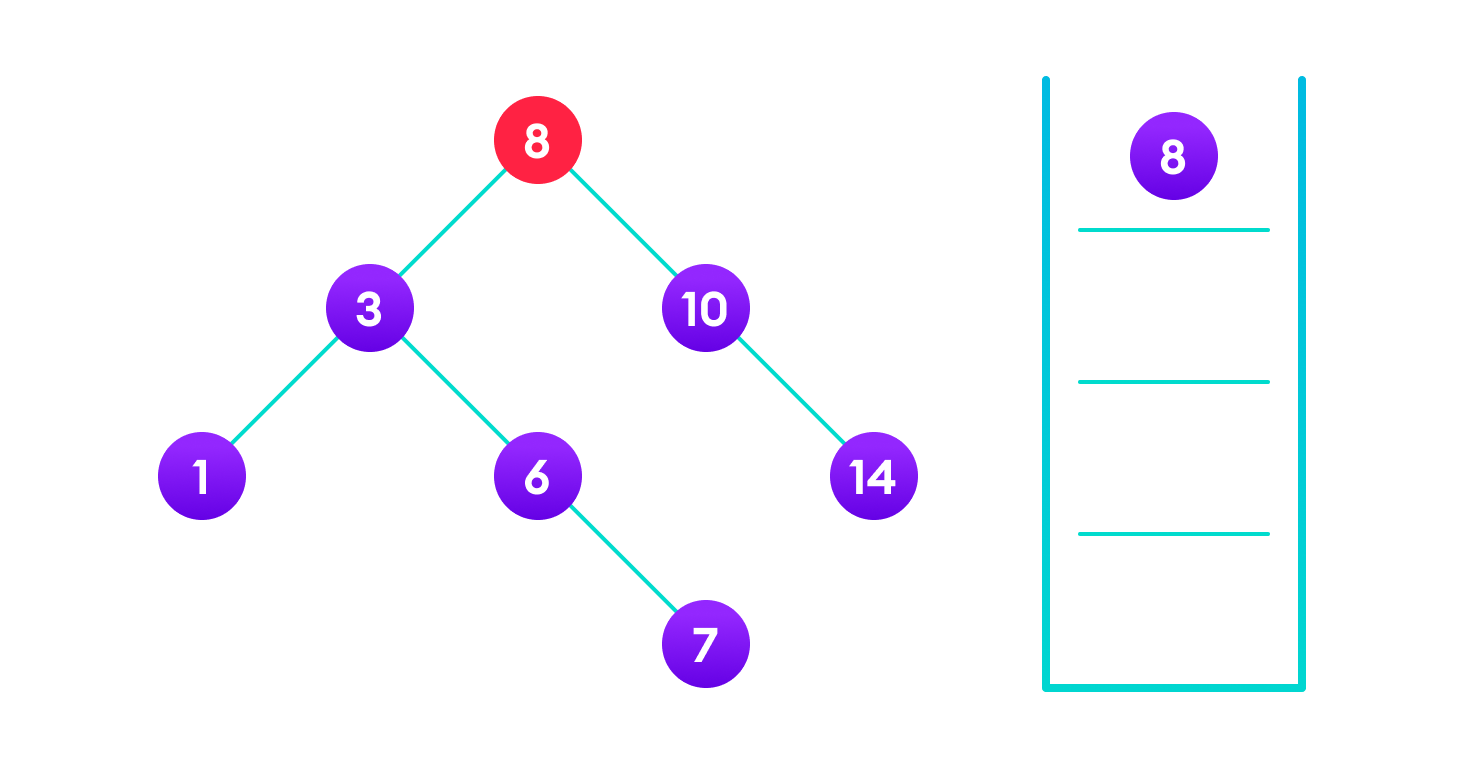4<8 so, transverse through the left child of 8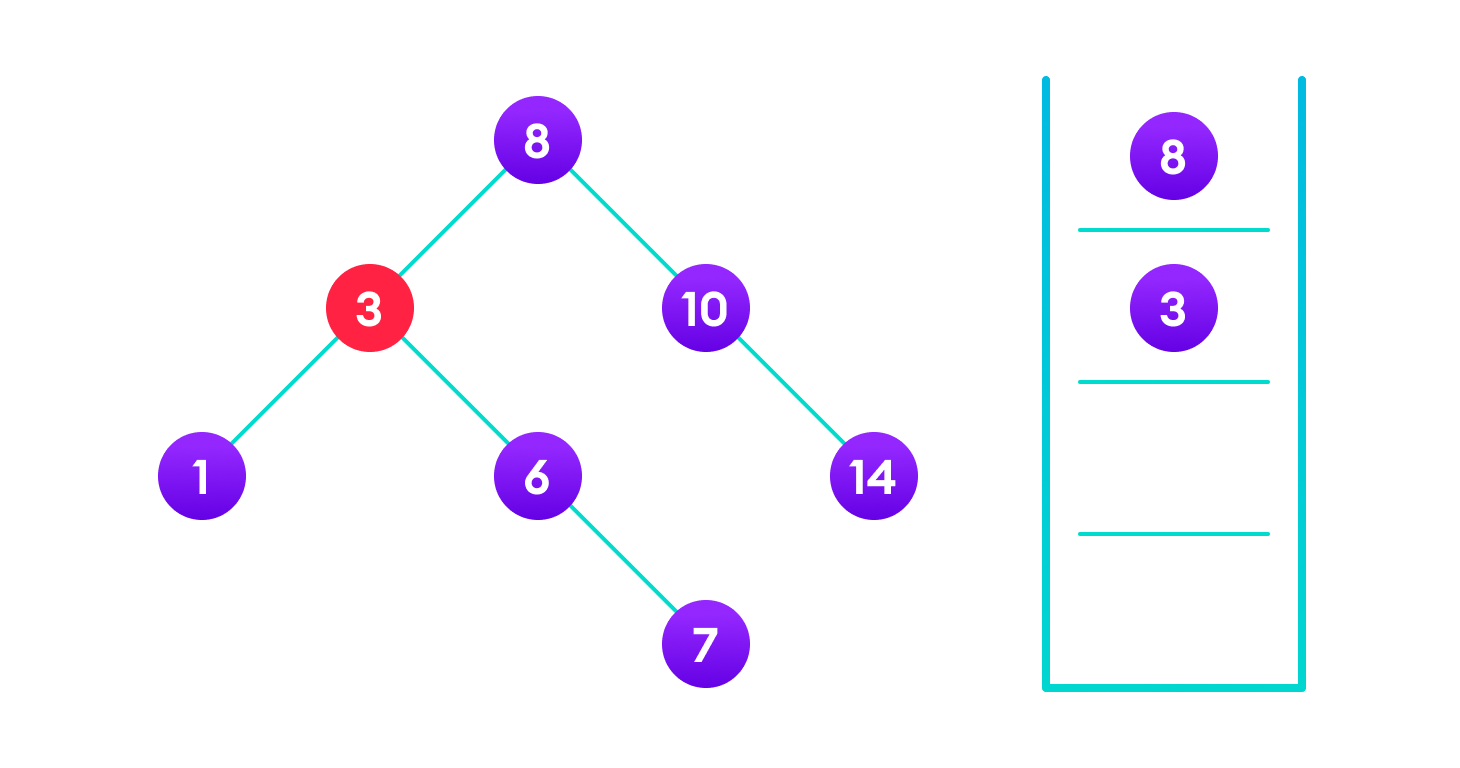4>3 so, transverse through the right child of 8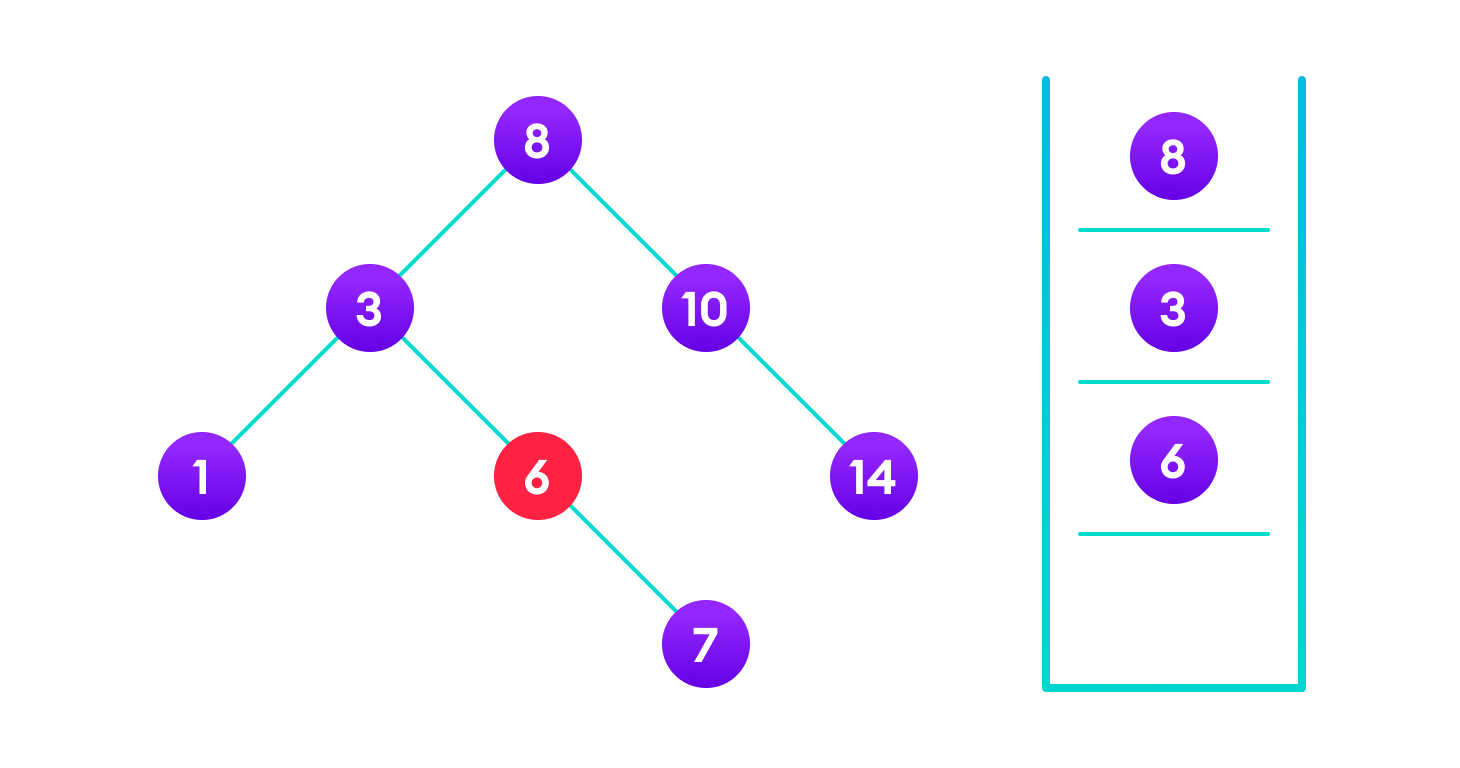4<6 so, transverse through the left child of 6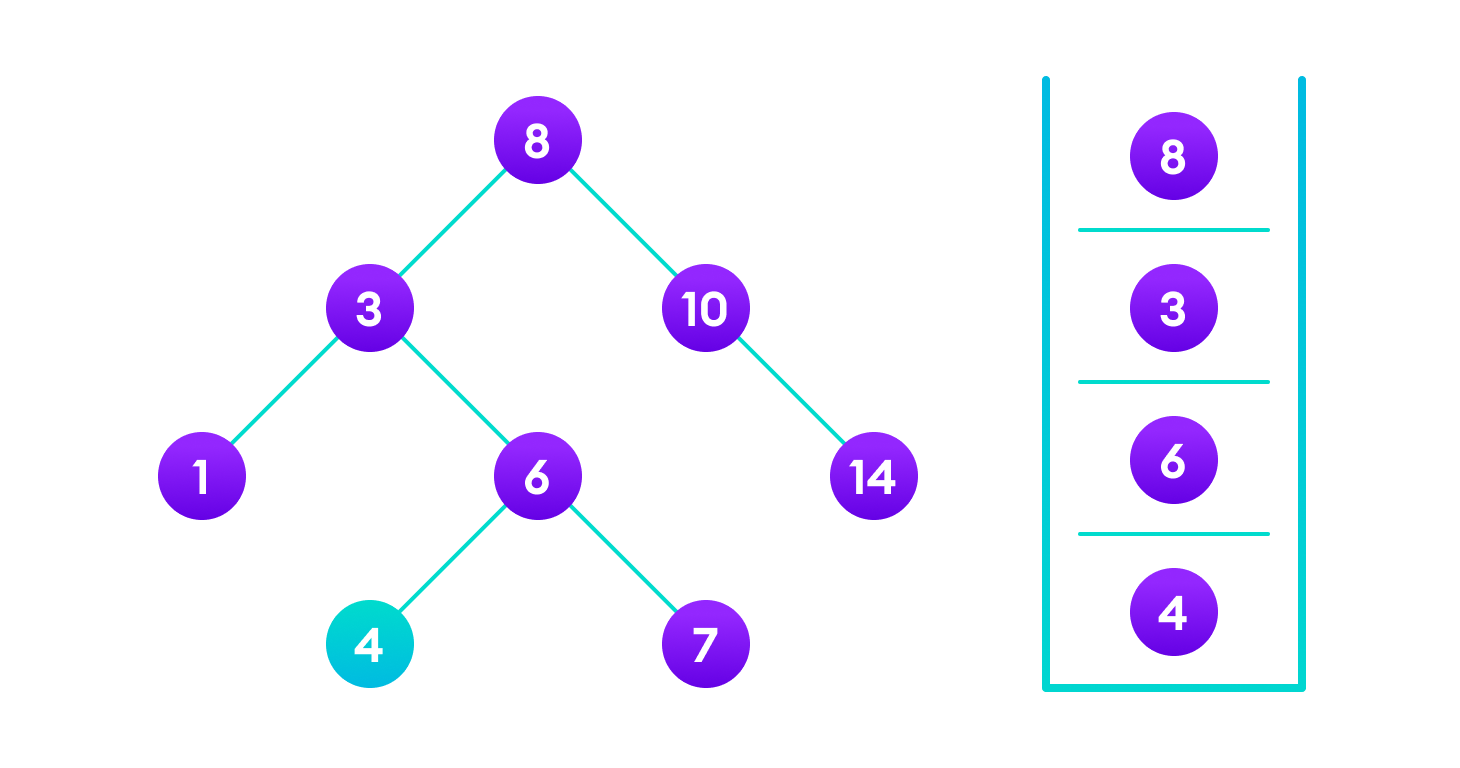Insert 4 as a left child of 6

We have attached the node but we still have to exit from the function without doing any damage to the rest of the tree. This is where the `return node;` at the end comes in handy. In the case of `NULL`, the newly created node is returned and attached to the parent node, otherwise the same node is returned without any change as we go up until we return to the root.

This makes sure that as we move back up the tree, the other node connections aren’t changed.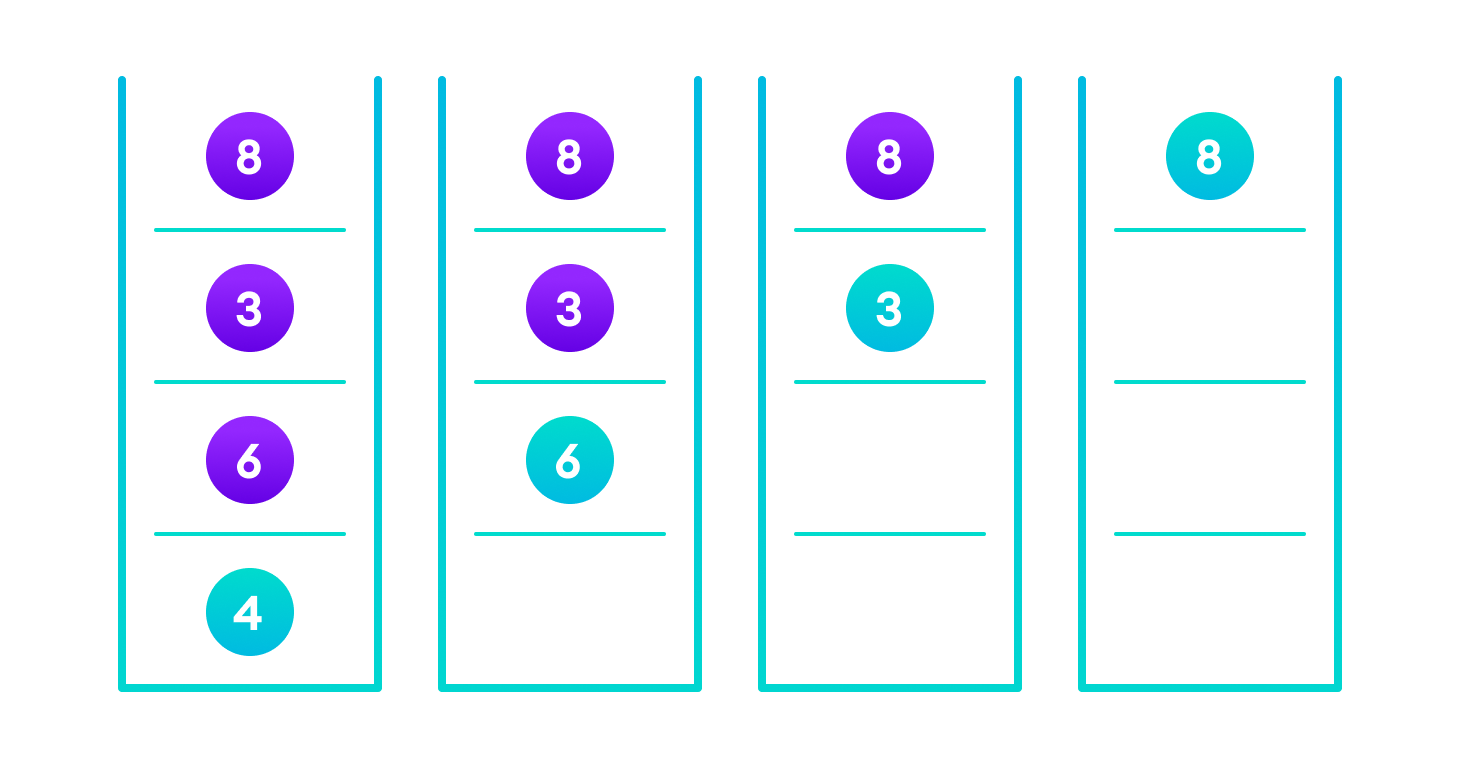Image showing the importance of returning the root element at the end so that the elements don’t lose their position during the upward recursion step.

## Deletion Operation

There are three cases for deleting a node from a binary search tree.

### Case I

In the first case, the node to be deleted is the leaf node. In such a case, simply delete the node from the tree.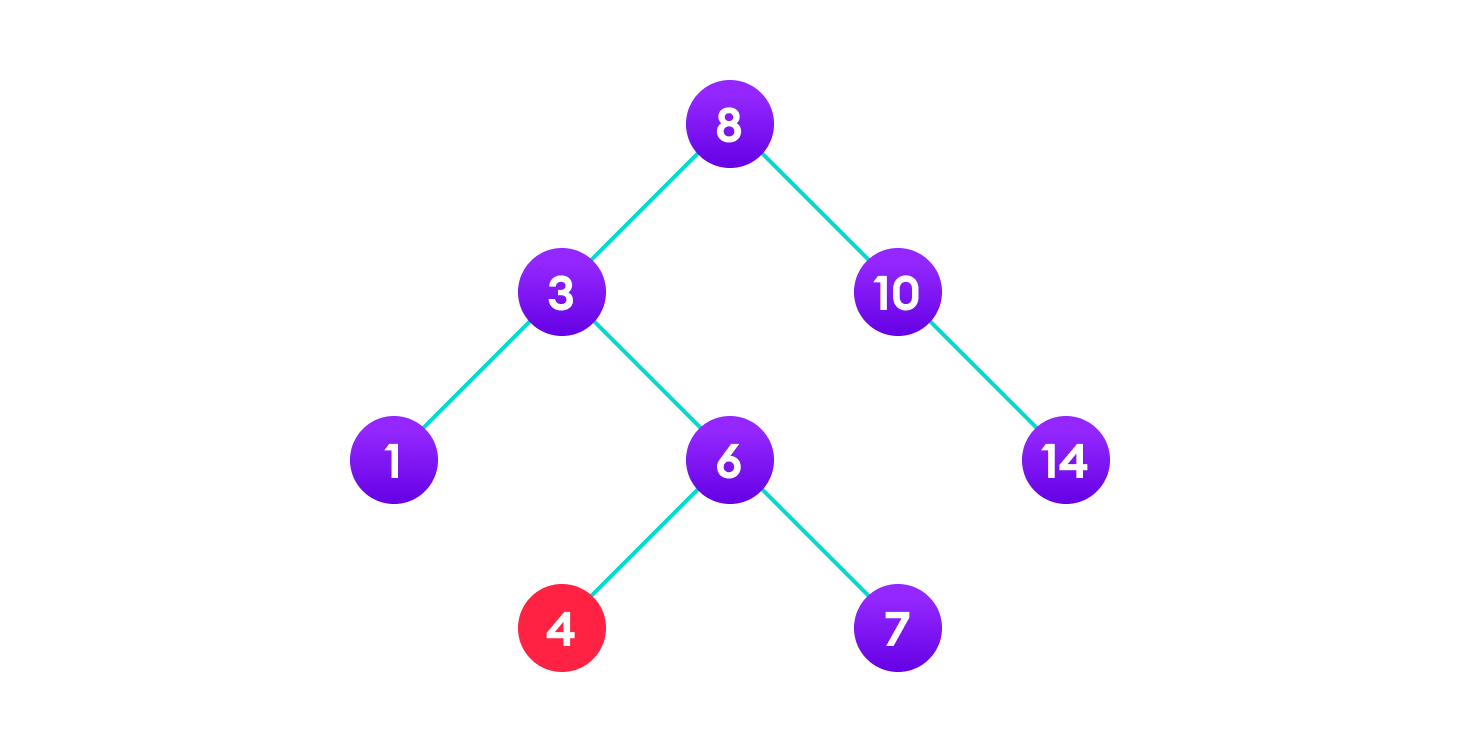4 is to be deleted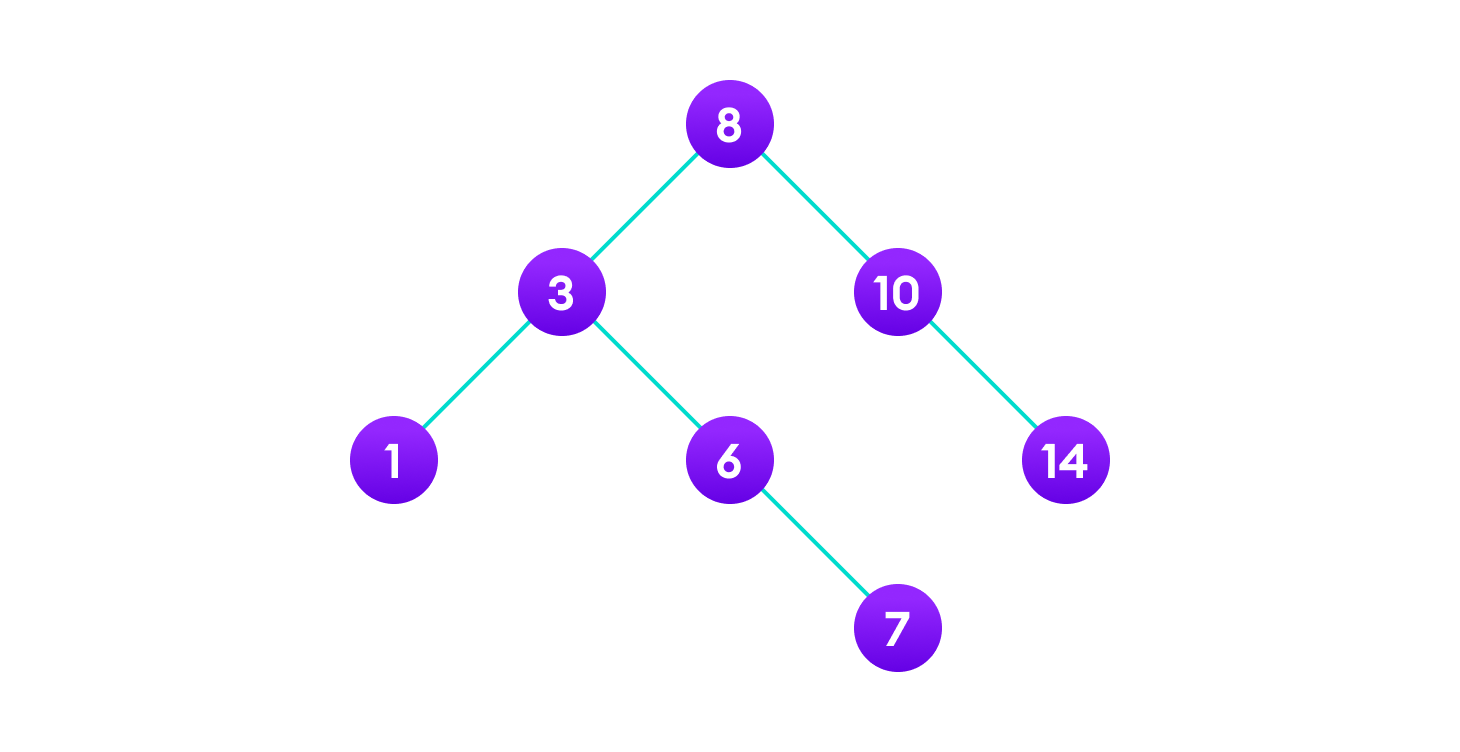Delete the node

### Case II

In the second case, the node to be deleted lies has a single child node. In such a case follow the steps below:

1. Replace that node with its child node.
2. Remove the child node from its original position.6 is to be deleted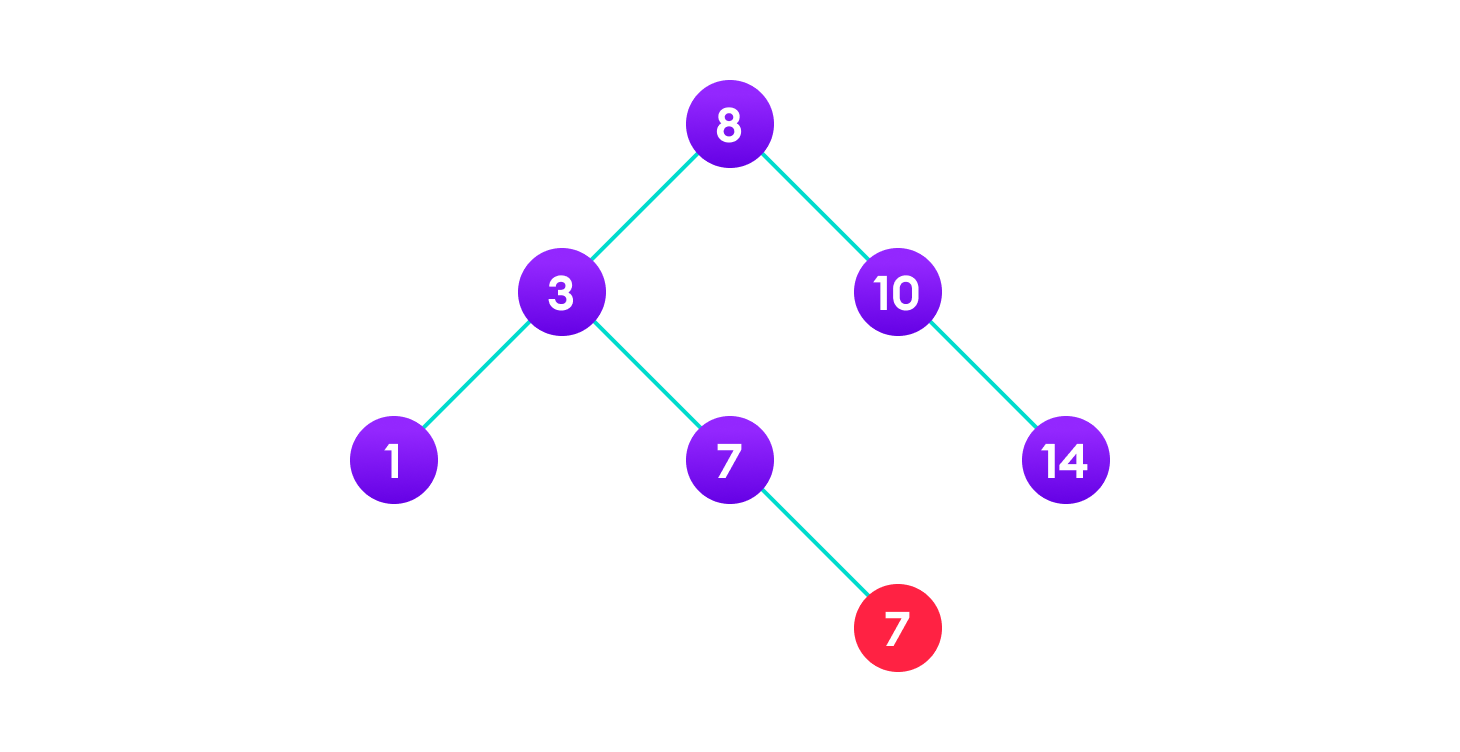copy the value of its child to the node and delete the child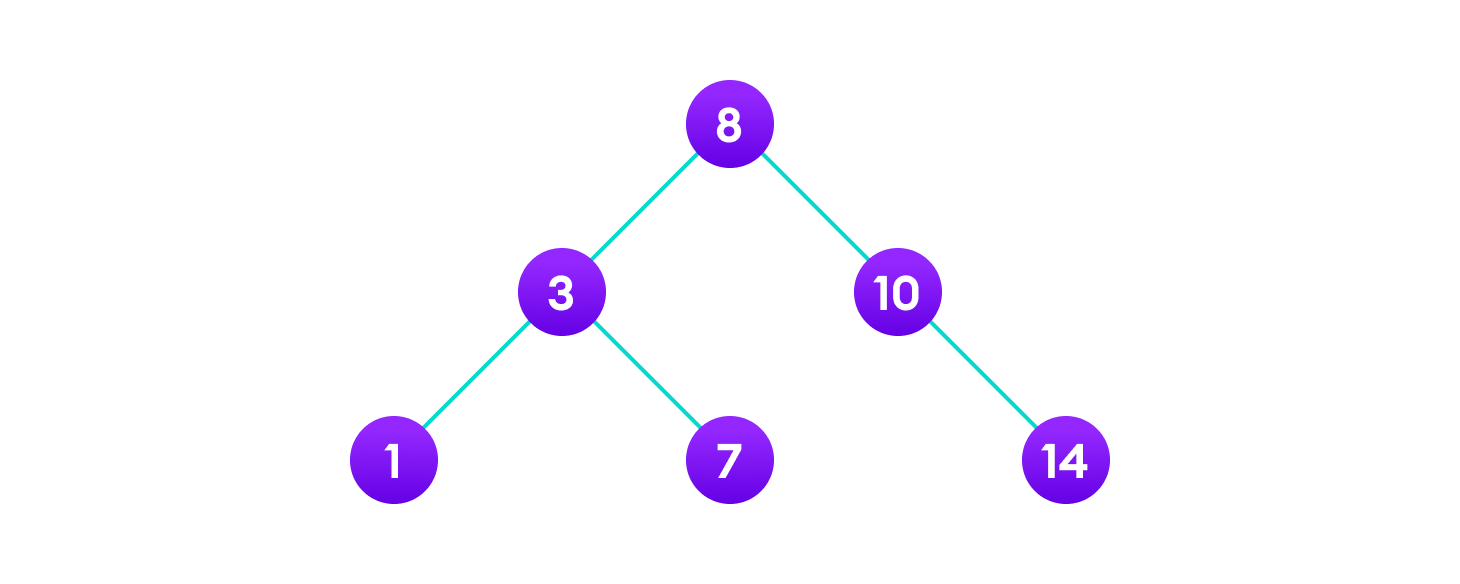Final tree

### Case III

In the third case, the node to be deleted has two children. In such a case follow the steps below:

1. Get the inorder successor of that node.
2. Replace the node with the inorder successor.
3. Remove the inorder successor from its original position.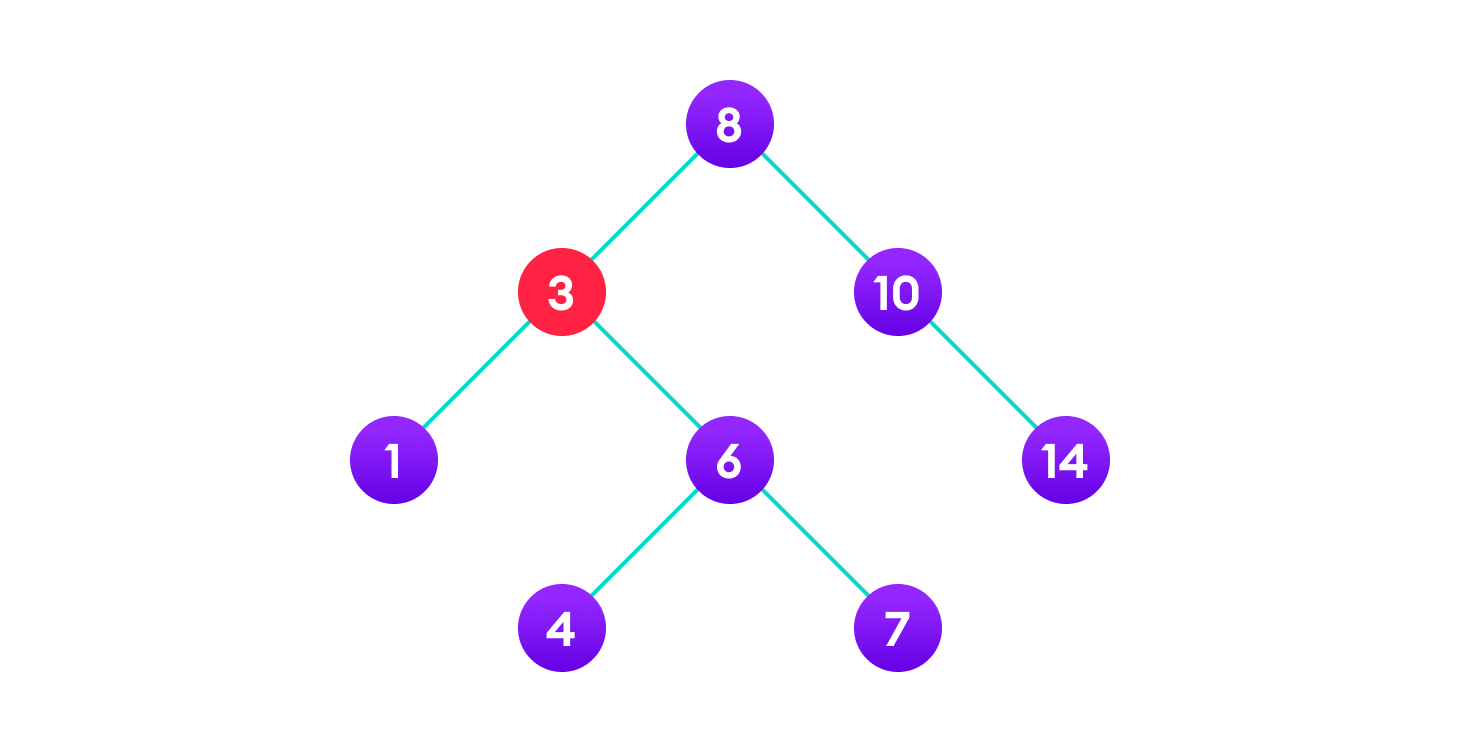3 is to be deletedCopy the value of the inorder successor (4) to the node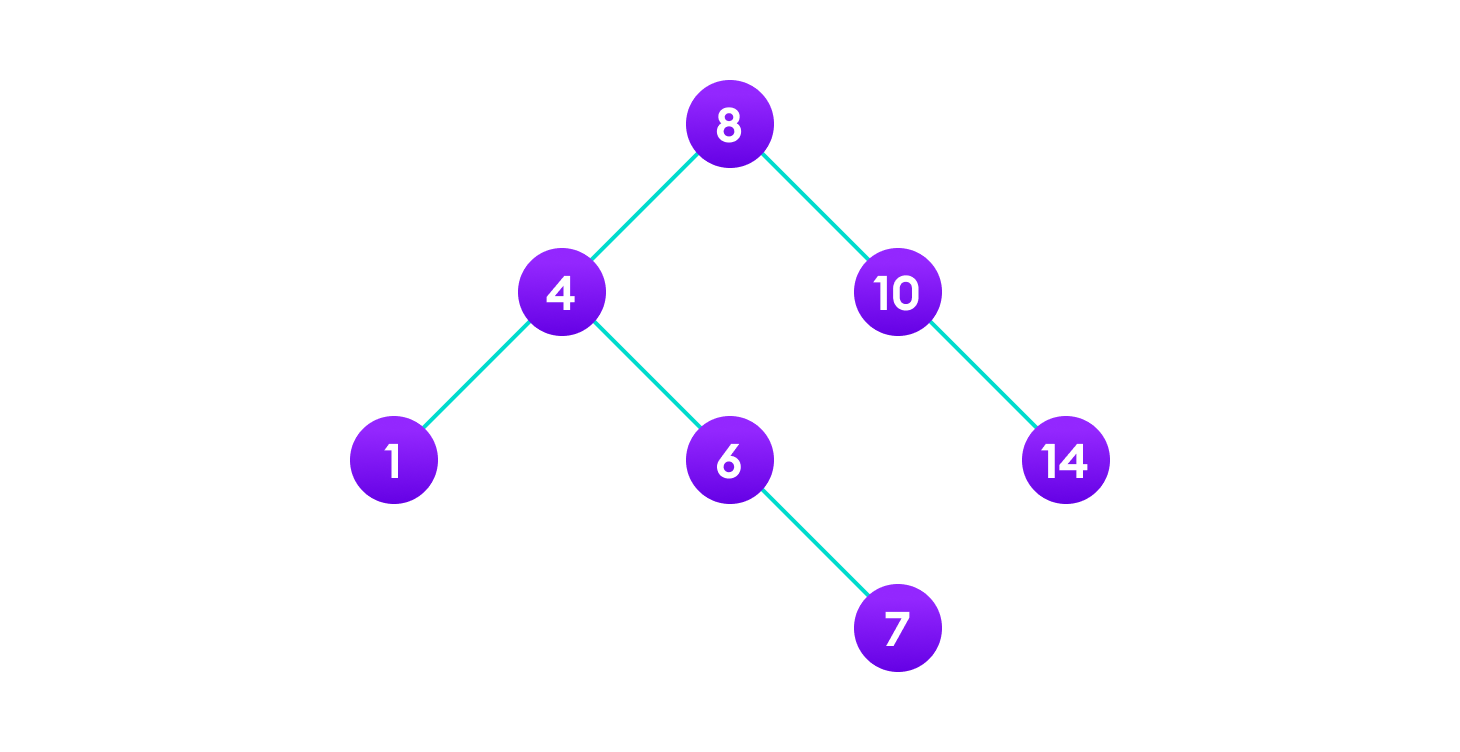Delete the inorder successor

## Python Examples

``````/* Binary Search Tree operations in Python */

/* Create a node */
class Node:
def __init__(self, key):
self.key = key
self.left = None
self.right = None

/* Inorder traversal */
def inorder(root):
if root is not None:
/* Traverse left */
inorder(root.left)

/* Traverse root */
print(str(root.key) + "->", end=' ')

/* Traverse right */
inorder(root.right)

/* Insert a node */
def insert(node, key):

/* Return a new node if the tree is empty */
if node is None:
return Node(key)

/* Traverse to the right place and insert the node */
if key < node.key:
node.left = insert(node.left, key)
else:
node.right = insert(node.right, key)

return node

/* Find the inorder successor */
def minValueNode(node):
current = node

/* Find the leftmost leaf */
while(current.left is not None):
current = current.left

return current

/* Deleting a node */
def deleteNode(root, key):

/* Return if the tree is empty */
if root is None:
return root

/* Find the node to be deleted */
if key < root.key:
root.left = deleteNode(root.left, key)
elif(key > root.key):
root.right = deleteNode(root.right, key)
else:
/* If the node is with only one child or no child */
if root.left is None:
temp = root.right
root = None
return temp

elif root.right is None:
temp = root.left
root = None
return temp

/* If the node has two children,
place the inorder successor in position of the node to be deleted */
temp = minValueNode(root.right)

root.key = temp.key

/* Delete the inorder successor */
root.right = deleteNode(root.right, temp.key)

return root

root = None
root = insert(root, 8)
root = insert(root, 3)
root = insert(root, 1)
root = insert(root, 6)
root = insert(root, 7)
root = insert(root, 10)
root = insert(root, 14)
root = insert(root, 4)

print("Inorder traversal: ", end=' ')
inorder(root)

print("nDelete 10")
root = deleteNode(root, 10)
print("Inorder traversal: ", end=' ')
inorder(root)``````

## Binary Search Tree Complexities

### Time Complexity

 Operation Best Case Complexity Average Case Complexity Worst Case Complexity Search O(log n) O(log n) O(n) Insertion O(log n) O(log n) O(n) Deletion O(log n) O(log n) O(n)

Here, n is the number of nodes in the tree.

### Space Complexity

The space complexity for all the operations is O(n).

## Binary Search Tree Applications

1. In multilevel indexing in the database
2. For dynamic sorting
3. For managing virtual memory areas in Unix kernel

# Special 95% discount

## 2000+ Applied Machine Learning & Data Science Recipes

### Portfolio Projects for Aspiring Data Scientists: Tabular Text & Image Data Analytics as well as Time Series Forecasting in Python & R## Two Machine Learning Fields

There are two sides to machine learning:

• Practical Machine Learning:This is about querying databases, cleaning data, writing scripts to transform data and gluing algorithm and libraries together and writing custom code to squeeze reliable answers from data to satisfy difficult and ill defined questions. It’s the mess of reality.
• Theoretical Machine Learning: This is about math and abstraction and idealized scenarios and limits and beauty and informing what is possible. It is a whole lot neater and cleaner and removed from the mess of reality.

Data Science Resources: Data Science Recipes and Applied Machine Learning Recipes

Introduction to Applied Machine Learning & Data Science for Beginners, Business Analysts, Students, Researchers and Freelancers with Python & R Codes @ Western Australian Center for Applied Machine Learning & Data Science (WACAMLDS) !!!

Latest end-to-end Learn by Coding Recipes in Project-Based Learning:

Applied Statistics with R for Beginners and Business Professionals

Data Science and Machine Learning Projects in Python: Tabular Data Analytics

Data Science and Machine Learning Projects in R: Tabular Data Analytics

Python Machine Learning & Data Science Recipes: Learn by Coding

R Machine Learning & Data Science Recipes: Learn by Coding

Comparing Different Machine Learning Algorithms in Python for Classification (FREE)

`Disclaimer: The information and code presented within this recipe/tutorial is only for educational and coaching purposes for beginners and developers. Anyone can practice and apply the recipe/tutorial presented here, but the reader is taking full responsibility for his/her actions. The author (content curator) of this recipe (code / program) has made every effort to ensure the accuracy of the information was correct at time of publication. The author (content curator) does not assume and hereby disclaims any liability to any party for any loss, damage, or disruption caused by errors or omissions, whether such errors or omissions result from accident, negligence, or any other cause. The information presented here could also be found in public knowledge domains.  `